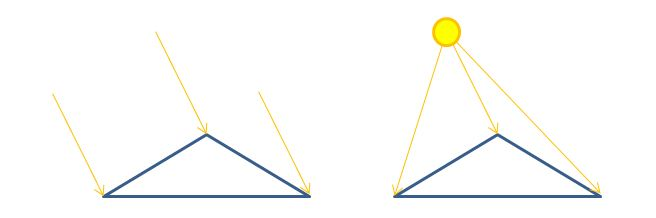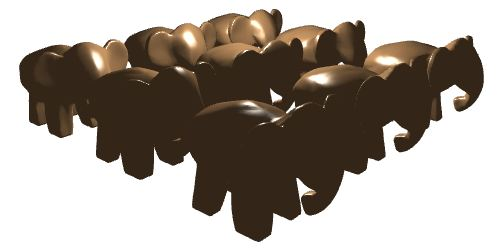Prev: Dir Lights per Pixel Next: Spotlights

When considering point lights, the main difference regarding directional lights  is that now we have a position for the light instead of a direction. This implies that the light direction is not constant across all fragments/vertices as it was for direction lights. Besides that, everything remains just like for directional lights. In the figure below we have a triangle being lit by a directional light (left) and a point light (right).The vertex shader now receives a light position instead of a light direction, and it must compute the light’s direction for each vertex. It is assumed that the light’s position is in camera space. Once we move the vertex position to the same space, the direction computation is straightforward:

light direction = light position – vertex position.

Before we can compute this vector we must apply the View Model matrix (`m_viewModel` in the shader) to the vertex position.

The new vertex shader is as follows:

```#version 330

layout (std140) uniform Matrices {
mat4 m_pvm;
mat4 m_viewModel;
mat3 m_normal;
};

layout (std140) uniform Lights {
vec4 l_pos;
};

in vec4 position;
in vec3 normal;

out Data {
vec3 normal;
vec3 eye;
vec3 lightDir;
} DataOut;

void main () {

vec4 pos = m_viewModel * position;

DataOut.normal = normalize(m_normal * normal);
DataOut.lightDir = vec3(l_pos - pos);
DataOut.eye = vec3(-pos);

gl_Position = m_pvm * position;
}```

The fragment shader is almost identical to the directional light case, the only difference being that it receives an interpolated light direction.

```#version 330

out vec4 colorOut;

layout (std140) uniform Materials {
vec4 diffuse;
vec4 ambient;
vec4 specular;
float shininess;
};

in Data {
vec3 normal;
vec3 eye;
vec3 lightDir;
} DataIn;

void main() {

vec4 spec = vec4(0.0);

vec3 n = normalize(DataIn.normal);
vec3 l = normalize(DataIn.lightDir);
vec3 e = normalize(DataIn.eye);

float intensity = max(dot(n,l), 0.0);
if (intensity > 0.0) {
vec3 h = normalize(l + e);
float intSpec = max(dot(h,n), 0.0);
spec = specular * pow(intSpec, shininess);
}

colorOut = max(intensity * diffuse + spec, ambient);
}```

An example image with a scene lit by a point light is shown below.Prev: Dir Lights per Pixel Next: Spotlights

### One Response to “GLSL Tutorial – Point Lights”

1.Hi, this line in the vertex shader

DataOut.eye = vec3(-pos);

could I ask why the pos is negated and what’s its purpose?

and the line in the fragment shader

vec3 h = normalize(l + e);

why adding l and e together? thank you

This site uses Akismet to reduce spam. Learn how your comment data is processed.